The EDIT_MESH application

Presentation

This application is controlled by the application menu (figure 1.32) on top of the screen and the selection menu (figure 1.20) at the bottom of the screen. It is used to edit a 2D mesh constructed from triangles and quadrangles, obtained either by switching from the CONSTRUCTION or PREP_MESH applications to the EDIT_MESH application, or by restoring a mesh (cf. general menus). Briefly, the possibilities are: adding, suppressing, moving the vertices of the mesh, reversing the edge that divides a quadrilateral, quadrangulating, triangulating, regularizing (placing the vertices at the barycenter of their neighbors), making the mesh Delaunay, modifying the references of a mesh (boundaries and subdomains), transforming a subdomain geometrically, renumbering, cracking.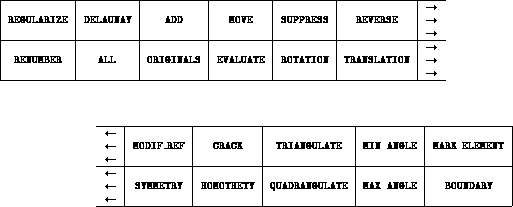Figure: The EDIT_MESH application menu

General remarks

The new types of selection of the application are:

• if the selection mode is ELEMENT, we select an element of type
 <ELEMENT>  which is a "finite element" (triangle  or quadrangle ) (just click in the finite element);

• if the selection mode is EDGE, we select an element of type <EDGE>  which is an edge of a mesh element (just click in the element that contains this edge or close to the latter);

• if the selection mode is VERTEX, we select an element of type <VERTEX>  which is a vertex of a mesh element (just click in the element that contains this vertex or close to the latter);

• if the selection mode is S_DOM, we select an element of type S_DOM  which is the connex component bounded by the interior and exterior boundaries and contains the clicked finite element.

Furthermore, we will often use the notation <point>  to denote a point <POINT>  , <COORD>  or <VERTEX>  .

<point> = <POINT> | <COORD> | <VERTEX>.

Definition of the state variables of the application

• MARK_ELEMENT   is used to visualize the elements whose angles are out of bounds (minimum and maximum angles for triangles and quadrangles).
• (TRIANGULATE|QUADRANGULATE) MIN_ANGLE  *<VALUE> is used to change the minimal value in degrees of angles for triangles or quadrangles.
• (TRIANGULATE|QUADRANGULATE) MAX_ANGLE  *<VALUE> is used to change the maximum value in degrees of angles for triangles or quadrangles.
• BOUNDARY  is used to move or not move the boundary points that are not extremities (flip flop). Initially, points cannot be moved with the MOVE  or REGULARIZE  operations.

Editing the mesh

The mesh editing operations are:

Moving a vertex

MOVE   *(<VERTEX>  <point>  |  BOUNDARY)
This command is used to move  the selected vertex (which can be on a boundary depending on the value of the state variable BOUNDARY) to the position of the selected point, which can either be a mouse_point (defined with the mouse), an xy_point (defined with two calculator values (x,y)), a DB point or a vertex (in the latter case, the new vertex is located at the cursor position and not on the selected vertex).
Remark: if we move a boundary point, the point moves on the boundary.

Adding a vertex to the mesh

ADD *(<VERTEX>  |  <ELEMENT>)
This command is used to add  a vertex in the mesh element that contains the selected point. If the selected element is a quadrangle, it is first split into two triangles. We add a vertex to the selected triangle by dividing it in three triangles. The coordinates of the new point are those of the cursor. Then the triangular mesh is made almost Delaunay.

Suppressing a vertex to the mesh

SUPPRESS  *(<VERTEX>  |  <S_DOM>)
This command is used to suppress :
1. either a non boundary point of the mesh and to remesh the hole thus created,
2. or a subdomain.

Reversing an edge of the mesh

REVERSE *( <EDGE>)

This command is used to reverse  the internal edge of the quadrilateral defined by two adjacent triangles, on the condition that the triangles thus created be correct.

Delaunay mesh

DELAUNAY  *(<ELEMENT> |  <S_DOM>  |  ALL )
This command is used to force the mesh to satisfy the Delaunay  criterion for triangles (the only vertices of the mesh contained in the circonscribed circle of a triangle are the vertices of this triangle),

1. either in the vicinity of the selected triangle,
2. or in the whole selected subdomain,
3. or in the whole mesh.

Regularizing the mesh

REGULARIZE  *(    <VERTEX>  |  <ELEMENT>  |  <S_DOM>
|   ALL |  ORIGINALS |  BOUNDARY)
This command is used to regularize the mesh or a part of it.   This means that we move all vertices of the part in question in turn to the barycenter of its neighbors. Boundary points are moved if this is permitted (cf. state variable).

Remark: if we move a boundary point, the point moves on the boundary.

Triangulating

TRIANGULATE *(  <ELEMENT>  |<S_DOM>  |   ALL |  ORIGINALS
| MIN_ANGLE  | MAX_ANGLE  )
This command is used to triangulate  the mesh or a part of it and to modify the minimum and maximum angle state variables of the triangles.

QUADRANGULATE    *(  <ELEMENT>   | <EDGE>  | <S_DOM>
|   ALL     |  ORIGINALS
| MIN_ANGLE  | MAX_ANGLE )
This command is used to ``quadrangulate"  the mesh or a part of it.

1. if an element is selected and if this elementĘis a triangle whose 3 adjacent elements are also triangles, then 3 quadrangles are created if these quadrangles are valid, i.e., convex and whose angles are between the minimum and maximum angles (cf. state variable).

2. if an edge is selected and if the 2 adjacent elements are triangles and if the edge is not the boundary of a subdomain, then a quadrangle formed from these 2 triangles is created if the quadrangle is valid.

3. if a subdomain is selected then the program will attempt to quadrangulate the entire subdomain (the method depends on the element selected to define the subdomain). Warning: the entire subdomain and even already existing quadrangles will be re-quadrangulated.

4. clicking the ALL  item or the ORIGINALS  item will cause the program to attempt quadrangulating the entire mesh (triangles might remain if the number of boundary edges is odd or if the angles are incorrect).

5. clicking the MIN_ANGLE  item (resp. MAX_ANGLE ) modifies the minimum angle state variable of the quadrangles (resp. maximum).

Cracking

CRACK *( <ARC> | <SEGMENT> | <SPLINE> )
This command is used to create or suppress cracks   in the mesh. If the selected element of the DB (arc, segment or spline) is cracked, then it will be ``uncracked", otherwise it will be cracked. A crack in a mesh is a line or a group of lines such that the nodes that are on one side or another of the line are different from each other. In the graphic representations the cracked nodes are separated from one another to demonstrate the crack. However, this is a purely graphic device.

Modifying the mesh references

MODIF_REF *( <VALUE>
*(  <ARC>    | <SEGMENT>  |  <SPLINE>
| <VERTEX> | <EDGE>    |  <S_DOM>)  )
This command is used to modify the references of different parts of the mesh.

Warning: the reference of a vertex is the reference of its supporting element in the DB (a point if it is an extremity) and similarly for edges. If the element is cracked, then there are two references, one on the left and the other one on the right.

Renumbering vertices

RENUMBER

This command is used to renumber the vertices of the mesh   in order to decrease the bandwidth or profile of the corresponding Lagrange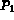or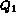finite element matrix.

The transformations

The transformations  apply either to subdomains or to the whole domain or only to the original subdomains, which is to say those that are drawn in continuous lines.
Note: a transformed, non original, subdomain is only defined by a pointer on the original subdomain and by the affine transformation (a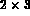matrix). This implies that all modifications, moving, adding, suppressing, etc., of the original subdomain will affect the transformed subdomains. Moreover, the original subdomains are displayed in continuous lines and the tranformed subdomains are displayed in dotted lines. To effectively generate all the transformed subdomains, we must click in the EVALUATE   box. The transformed subdomains then become original subdomains since we have evaluated the expression that defines the mesh.

Warning: as long as the tranformed subdomains are not evaluated, the DB boundary elements for these subdomains do not exist yet.

A point point will either denote a vertex or a mouse point, an xy point or a current point. The syntax is:

<a_transformation> ( ALL |  ORIGINALS | <S_DOM>*)
This means that after it is defined, a transformation will either apply to all subdomains or to the original subdomains   (in continuous lines) or to explicitly selected subdomains.

A transformation is either a translation, a symmetry, a rotation or a homothety. Syntactically:

<a_transformation>  =   <translation>  |  <symmetry>
| <rotation>    |  <homothety>;

which are defined as follows:

Translation

<translation>  = TRANSLATION   <point>  <point>
Defines the translation  going from the first point to the second point.

Symmetry

<symmetry>  = SYMMETRY  (   <point_coor>  <point_coor>
| <SEGMENT>  | <EDGE>)
Defines the symmetry  with respect to the straight line that supports the selected segment or edge, or that passes through the two selected points.

Rotation

<rotation>  = ROTATION (<point>  <VALUE>  |  <VALUE>  <point>)

Defines the rotation  around the point and with angle equal to the value entered on the calulator.

Homothety

<homothety>  = HOMOTHETY  (<point> <VALUE> | <VALUE> <point>)
Defines the homothety  of center the point and ratio equal to the value.

Effectively generating the transformations

EVALUATE

This command is used to effectively generate   all the transformed subdomains in order to edit them independently of their original. It also creates and transforms all the elements (segments, arcs, splines) that are referenced by the transformed subdomains.

An example of transformation

To create symmetrical subdomains we click in the SYMMETRY item, then we select either two points, a segment or an edge, then we select either all the subdomains to be transformed, or we click in the ALL item or in the ORIGINALS item. Finally, before saving the mesh under another format than "mesh", we click in the EVALUATE item.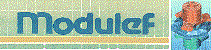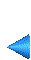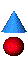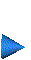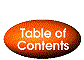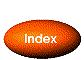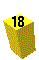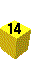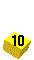Next: 1.5.6 Example Up: The EMC˛ preprocessor Prev: The PREP_MESH application Index Contents